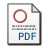# An Analysis Model for the Disturbances Generated by Collinearity in the Context of the OLS Method

by Pavelescu, Florin Marius
Published in Romanian Journal of Economic Forecasting
, 2010, volume 13 issue 2, 245-264Requires a PDF viewer such as Xpdf or Adobe Acrobat Reader  144Kb

## Abstract

Under the conditions of OLS use in order to perform multiple linear regressions, both the estimated parameters values and also computed values of some statistical tests such as coefficient of determination, Fisher test or Student test are influenced by collinearity1. The respective influence is revealed by the values of the coefficient of alignment to collinearity hazard. For this reason, this paper presents an analysis model which identifies the factors and their influences on the above-mentioned indicator. On the one hand, we quantify the factors contribution to the arithmetical mean of coefficients of alignment to collinearity hazard, having in view that the respective indicator reveals the collinearity impact on a linear regression model as a whole. On the other hand, we emphasize the necessary conditions for the positivity of all coefficients of alignment to collinearity hazard, in order to avoid the occurrence of “unexpected signs” in case of some estimated parameters. Also, we bring some clarifications and extensions of the other concepts previously proposed by the author such as: the main and secondary explanatory variable, coefficient of mediated by resultative variable correlation between explanatory variables.

Keywords: arithmetical mean of coefficients of allignament to collinearity hazard, main  explanatory variables, secondary explanatory variables, coefficient of correlation between  explanatory variables mediated by resultative variable, structural constraints of a multiple  linear regression model.
JEL Classification:
C13, C20, C51, C52